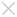### Suggestions

Home Page ZW3D Tips & Tricks Design Variable Pitch Helix by Equation Curve

## Learning

• ### White Paper

#### Design Variable Pitch Helix by Equation Curve

A variable pitch helix is often used in product design, especially in mechanicalproducts. In ZW3D, designers can easily create various helixes by using the Equation Curve feature.

Here are some existing equations that are used to create helical curves. Firstly, let’s see the simplest helix with constant radius and constant pitch.

The table below shows the general expression, two different methods.

 Cylindrical coordinates Cartesian coordinates r(t) = Radius     theta(t) = 360*Num_turns*t     z(t) = Num_turns*Pitch*t x(t) = Radius*sin(360*Num_turns*t)     y(t) = Radius*cos(360*Num_turns*t)     z(t) = Num_turns*Pitch*tt∈（0,1）To make the variable pitch, just replacePitch with Pitch*t.

Take a look at other spiral curves.

(R mans radius; P means pitch)

 Name Equation Spiral curve Spiral curve (constant R, variable P)Spiral curve (variable R,constant  P)Spiral curve (variable R,   variable P)In real design, some spiral curves are irregular, meaning designers couldn’t directly use these existing equation curves. Don’t worry! You can now modify the equation to get your required spiral curves.

Firstly,Let’see if it is possible to use a general expression to cover all of the above cases.r(t) =R1 + R2 * t

thera(t) = 360 * N * tz(t) = N * P1 * t + N * P2 * t * tWhen R2 and P2 are both 0, this sprial curve is a general spiral helix curve. If R1 and P1 are both 0 , this is a variable radius & variable pitch spiral curve.

So by using this general expression, designers can work more productively!

For example, there is a spiral curve with constant radius (50mm),variable pitch (from 20 to 60mm) and length of 200mm.

According to these given conditions and the general expression, we get the following equations:

200 = N*mean-pitch = N*{(Start-pitch+End-pitch)/2} = N* {(20+60)/2}= N * 40

20 = Start-pitch(threa=360) = Start-pitch(N*t=1) = P1 + P2*t = P1 +(P2/N)

200 = Z(t=1) = N*P1 + N*P2

The result of variables:  N= 5;  P1= 15;  P2=25;

So the expression of this spiral curve is：

r(t) = 50

thera(t) = 360 * 5 * t

z(t) = 5* 15* t + 5* 25 * t * tShort Description

ZW3D provides many different equation curves, including several different types of spiral curve. Comprehensively understanding the meaning of each parameter, designers are able to use the equation to create their desired curves. How to deisgn variable pitch helixs by equation curve is introduced in this article.

Key words:

ZW3D, equation curve, helix curve, spiral curve, variable pitch helix

ZW3D is all-in-one, affordable CAD/CAM which enables concept to finished product design in an integrated, collaborative environment. The proprietary Overdrive™ kernel delivers 3D part and assembly modeling, 2D production drawings, reverse engineering, motion simulation, mold design and integrated CNC machining, simplifying the design process from concept to completion. Experience ZW3D 2013, where the only limit is your imagination. Please go to www.zwsoft.comto download a free 30-day trial today.

http://www.zwsoft.com

Author’s Name:

Author’s Info:

Orchid Jin

• Previous

• Next

## ·ZW3D 2016 Know-how:How to Use the Upgraded Pattern Command?2016-03-31